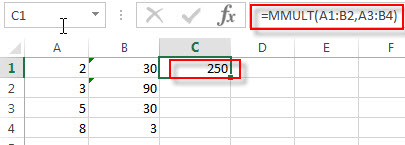# Excel MMULT Function

This post will guide you how to use Excel MMULT function with syntax and examples in Microsoft excel.

### Description

The Excel MMULT function returns the matrix product of two arrays.

If you want to return multiple result in an array on the worksheet, then you need to input an array formula in the MMULT function.

To input array formulas, you need to highlight the range of cells that this function is to be entered into, and then type the function into the first cell of the range, and press shortcuts Ctrl + Shift + Enter.

And the returned array result will contain the same number of rows as array1 and the same number of columns as array2.

The MMULT function is a build-in function in Microsoft Excel and it is categorized as a Math and Trigonometry Function.

The MMULT function is available in Excel 2016, Excel 2013, Excel 2010, Excel 2007, Excel 2003, Excel XP, Excel 2000, Excel 2011 for Mac.

### Syntax

The syntax of the MMULT function is as below:

`=MMULT (array1,array2)`

Where the MMULT function arguments are:

• array1 – This is a required argument. The first array that you wish to multiply.
• array2 – This is a required argument. The second array that you wish to multiply.

Note:

• The array can be a cell range or an array constant, such as: {2,3;5,6} and both arrays must contain only numeric values.
• If any cells in array are empty or contain text string, then the MMULT function will return the #VALUE! Error.
• If the Array does not have the same number of rows and columns, then the MMULT function will return the #VALUE! Error.
• Formulas that return arrays must be entered as array formulas.

### Excel MMULT Function Examples

The below examples will show you how to use Excel MMULT Function to calculate the matrix product of two arrays.

1# to calculate the matrix product of two arrays A1:B2 and A3:B4, enter the following formula in Cell D1.

`=MMULT(A1:B2,A3:B4)`### Related Functions

• Excel MDETERM function
The Excel MDETERM function returns the matrix determinant of an array. So you can use the MDETERM function to calculate the determinant of a square matrix in Excel.The syntax of the MDETERM function is as below:=MDETERM(array)…
• Excel MINVERSE Function
The Excel MINVERSE function returns the inverse matrix for a given matrix.The syntax of the MINVERSE function is as below:=MINVERSE(array)…